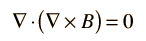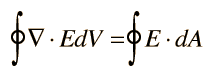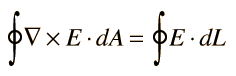# Vector Calculus Identities

The divergence of the curl is equal to zero:The curl of the gradient is equal to zero:More vector identities
Index

Vector calculus

 HyperPhysics*****HyperMath*****Calculus R Nave
Go Back

# Divergence Theorem

The volume integral of the divergence of a vector function is equal to the integral over the surface of the component normal to the surface.Index

Vector calculus

 HyperPhysics*****HyperMath*****Calculus R Nave
Go Back

# Stokes' Theorem

The area integral of the curl of a vector function is equal to the line integral of the field around the boundary of the area.Index

Vector calculus

 HyperPhysics*****HyperMath*****Calculus R Nave
Go Back

# Vector Identities

In the following identities, u and v are scalar functions while A and B are vector functions. The overbar shows the extent of the operation of the del operator.Index

Vector calculus

 HyperPhysics*****HyperMath*****Calculus R Nave
Go Back